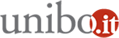# Probability and Stochastic Processes

Linda Altieri

Junior Assistant Professor

• Environmental statistics
• Bayesian inference
• Point processes
• Entropy measures

Alessandro Baldi Antognini

Professor

• Sequential designs for comparative experiments
• Statistical applications to clinical trials
• Optimal design theory
• Markov chains

Enrico Bernardi

Professor

• Nonlinear ordinary differential equation-Cauchy problem for hyperbolic PDEs-stability for stochastic Hamiltonian systems- combinatoric copula-based algorithms

Maurizio Brizzi

Associate Professor

• Archaeological Statistics
• Indices of variability and shape derived from quantiles
• Risk indices defined by applying fuzzy procedures
• Probabilistic methods applied to hazard games
• Statistical analysis of alternative medicine experiments

Maria Letizia Guerra

Associate Professor

• Interval analysis in decision science
• Fuzzy sets - non-additive uncertainty theory like possibility theory

Alberto Lanconelli

Assistant Professor

• Foundational and modelling issues of the theory of stochastic differential equations-numerical approximations and infinite dimensional Gaussian analysis

Alessandra Luati

Professor

• Locally stationary stochastic processes
• Frequency domain analysis
• Score driven models

Sabrina Mulinacci

Associate Professor

• Reneralizations of the Marshall-Olkin distribution for credit risk modeling and joint life modeling in insurance- copula based AR(1) processes for non-rational expectations
• Generalization of the Marshall-Olkin distribution- copula based stochastic processes in discrete times

Daniele Ritelli

Associate Professor

• Ordinary differential equations- elliptic functions and integrals
• Special functions
• Bessel and hypergeometric

Silvia Romagnoli

Associate Professor

• Credit risk models- optimal hedge ratio- spectral risk measure-pricing and ambiguity
• Combinatoric copula-based algorithms-skew distributions- copulas and stochastic processes- measure invariance of copulas

Maroussa Zagoraiou

Associate Professor

• Sequential designs for comparative experiments
• Statistical applications to clinical trials
• Optimal design theory
• Markov chains